Next: Free Form Q Up: Examples Previous: Examples

## Fixed form Q

Let us model a set of observations,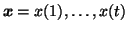, by a Gaussian distribution parametrised by mean m and log-std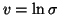. We shall approximate the posterior distribution by q(m,v) = q(m)q(v), where both q(m) and q(v) are Gaussian. The parametrisation with log-std is chosen because the posterior of v is closer to Gaussian than the posterior of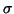or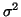would be. (Notice that the parametrisation yielding close to Gaussian posterior distributions is connected to uninformative priors discussed in section 5.1.)

Let the priors for m and v be Gaussian with means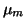and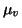and variances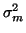and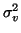, respectively. The joint density of the observations and the parameters m and vis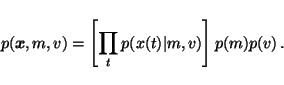(19)

As we can see, the posterior is a product of many simple terms.

Let us denote by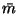and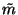the posterior mean and variance of m.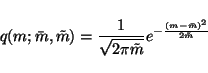(20)

The distribution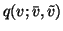is analogous.

The cost function is now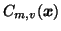=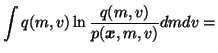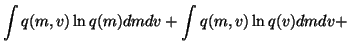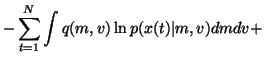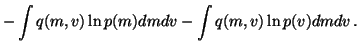(21)

We see that the cost function has many terms, all of which are expectations over q(m, v). Since the approximation q(m, v) is assumed to be factorised into q(m, v) = q(m) q(v), it is fairly easy to compute these expectations. For instance, integrating the term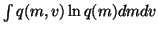over v yields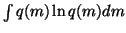, since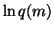does not depend on v and. Since q(m) is assumed to be Gaussian with meanand variance, this integral is, in fact, minus the entropy of a Gaussian distribution and we have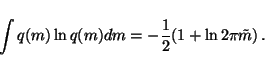(22)

A similar term, withreplaced by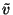, comes from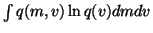.

The terms where expectation is taken over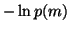and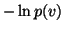are also simple since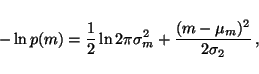(23)

which means that we only need to be able to compute the expectation of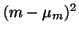over the Gaussian q(m) having meanand variance. This yields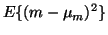=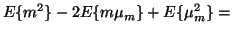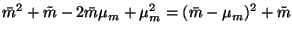(24)

since the variance can be defined by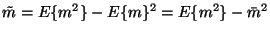which shows that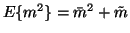. Integrating the equation 23 and substituting equation 24 thus yields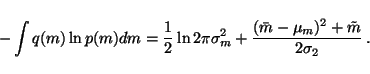(25)

A similar term, with m replaced by v, will be obtained from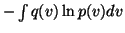.

The last terms are of the form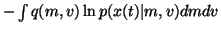. Again we will find out that the factorisation q(m, v) = q(m) q(v) simplifies the computation of these terms. Recall that x(t)was assumed to be Gaussian with mean m and variance e2v. The term over which the expectation is taken is thus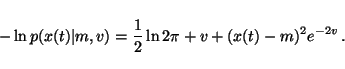(26)

The expectation over the term (x(t) - m)2 e-2v is easy to compute since m and v are assumed to be posteriorly independent. This means that it is possible to take the expectation separately from (x(t) - m)2 and e-2v. Using a similar derivation as in equation 24 yields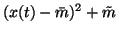for the first term. The expectation over e-2v is also fairly easy to compute: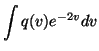=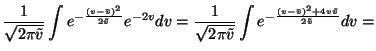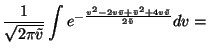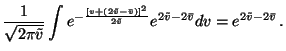(27)

This shows that taking expectation over equation 26 yields a term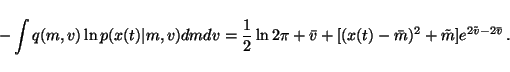(28)

Collecting together all the terms, we obtain the following cost function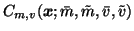=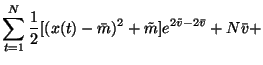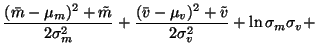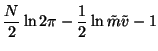(29)

Assumingandare very large, the minimum of the cost function can be solved by setting the gradient of the cost function C to zero. This yields the following:=(30)=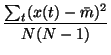(31)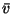=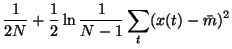(32)=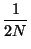(33)

In caseandcannot be assumed very large, the equations forandare not that simple, but the solution can still be obtained by solving the zero-point of the gradient.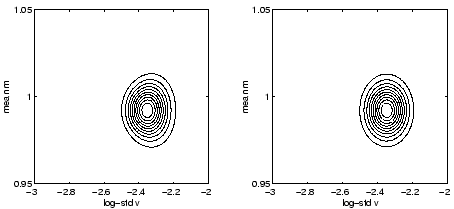Figure 3 shows a comparison of the true posterior distribution and the approximate posterior. The data set consisted of 100 points drawn from a model with m=1 and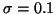. The contours in both distributions are centred in the same region, a model that underestimates m.The contours for the two distributions are qualitatively similar although the true distribution is not symmetrical about the mean value of v.Next: Free Form Q Up: Examples Previous: Examples
Harri Lappalainen
2000-03-03